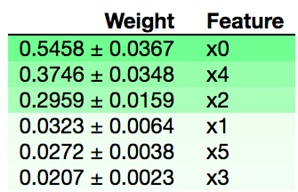﻿ Using scikit-learn Permutation Importance to Weight the Importance of Variables in a Regression Model of the US Election – BMC Blogs
Machine Learning & Big Data Blog

Using scikit-learn Permutation Importance to Weight the Importance of Variables in a Regression Model of the US Electionby

This is going to be an interesting post, because we show why people in the last US election voted for Trump, which is the same as saying against Clinton, since the fringe candidates hardly got any votes, relatively speaking.

The technique here handles one of the most vexing problems in black box classifier and regression models, which is which variables should you remove from a regression model to make them more accurate.

Permutation Importance, how it Works

We use the Permutation Importance method of the ELI5 scikit-learn Python framework. The downside to this is (1) other ML frameworks do not support this and (2) its output is an HTML object that can only be displayed using iPython (aka Jupyter).

Permutation Importance works for many scikit-learn estimators. It shuffle the data and the removes different input variables to see what relative change results in the calculating the training model. It measures how much the outcome goes up or down given the input variable, thus calculating their impact on the results.

In other words, for linear regression, it first calculates, for example, the coefficients α, β, γ, …

`y = αa + βb + γc + … + ωz + B`

And then tests the model using cross entropy or some other technique and then calculating r2 score, F1, and accuracy then drops one of a, b, c, … and runs it again.

Running Jupyter

Nothing can be easier that running Jupyter, meaning it is easier to set up that Zeppelin, however Zeppelin does not require much set up at all. You just install Anaconda and then, on Mac, type jupyter notebook. It will open this URL in the browser http://localhost:8889/tree.

Clinton v. Trump

We get US election data here. These are summaries by county for each state in the US. The code we write we store here.

We use the read_csv Pandas method to read the election data, taking only a few of the columns. You could add more columns to find what other variables correlate with the voter’s choice.

At the bottom is the complete code. Here we explain different sections. But first, here are the results in both HTML and text format.

So you can see the columns in the data frame by their index, here they are are:

```Index(['Trump', 'age65plus', 'SEX255214', 'White', 'Black', 'Hispanic',
'Edu_highschool'],
dtype='object')```

Here here are the relative weights.

 column name Weight Feature age65plus 0.5458 ± 0.0367 x0 Hispanic 0.3746 ± 0.0348 x4 White 0.2959 ± 0.0159 x2 SEX255214 0.0323 ± 0.0064 x1 Edu_highschool 0.0272 ± 0.0038 x5 Black 0.0207 ± 0.0023 x3

The graphic is shown in the iPython notebook as follow:So you can see, the decision whether or not to vote for Trump is mainly by age, with voters 65 and over most closely correlated to the outcome. Surprisingly the sex does not matter much at all.

The Code Explained

In another blog post we explain how to do linear regression. The technique is the same here, except we use more than one independent variable, i.e., x.

We take as the independent variables xx, everything but Trump, which is the dependant variable, yy. And a vote for Trump is a vote not for Hillary. So the output for the yy variable should the same, or similar. (You can check that out). But it’s not going to be exactly the same as yy <> 1 – xx in the data.

We use the values properties of the dataframe to convert that to a numpy array as that it what the fit method of LR requires..

```xx = train[['White','Black','Hispanic','age65plus','Edu_highschool','SEX255214']].values
yy = train[['Trump']].values```

We do not need to reshape the arrays as the dimensions fit the requirement that they can be paired up. xx has 3112 rows nd 6 columns. yy is 3112 x 1.

```xx.shape is (3112, 6)
yy.shape is (3112, 1)```

Next we run the fit method of linear_model. Then we print the coefficients:

```Coefficients:
[[ 0.51503519 -0.13783668 -0.44485146  0.00352575 -0.00985589 -0.00801731]]```

Then comes the grand finale which is to run the fit method of PermutationImportance then draw the graph. As we mentioned before it shuffles the input variables and runs linear regression multiple times and then calculates which independent variables have the greatest impact on the calculation of the coefficients and thus y,

```perm = PermutationImportance(reg, random_state=1).fit(xx, yy)
eli5.show_weights(perm)```

Here is the complete code.

```import matplotlib.pyplot as plt
from sklearn import linear_model
import numpy as np
from sklearn.metrics import mean_squared_error, r2_score
import pandas as pd
import eli5
from eli5.sklearn import PermutationImportance

xx = train[['White','Black','Hispanic','age65plus','Edu_highschool','SEX255214']].values
yy = train[['Trump']].values
reg = linear_model.LinearRegression()
model=reg.fit(xx,yy)
print('Coefficients: \n', reg.coef_)
perm = PermutationImportance(reg, random_state=1).fit(xx, yy)
eli5.show_weights(perm)```

Wikibon: Automate your Big Data pipeline

Learn how data management experts throughout the industry are transforming their Big Data infrastructure for maximum business impact.

These postings are my own and do not necessarily represent BMC's position, strategies, or opinion.

See an error or have a suggestion? Please let us know by emailing blogs@bmc.com.